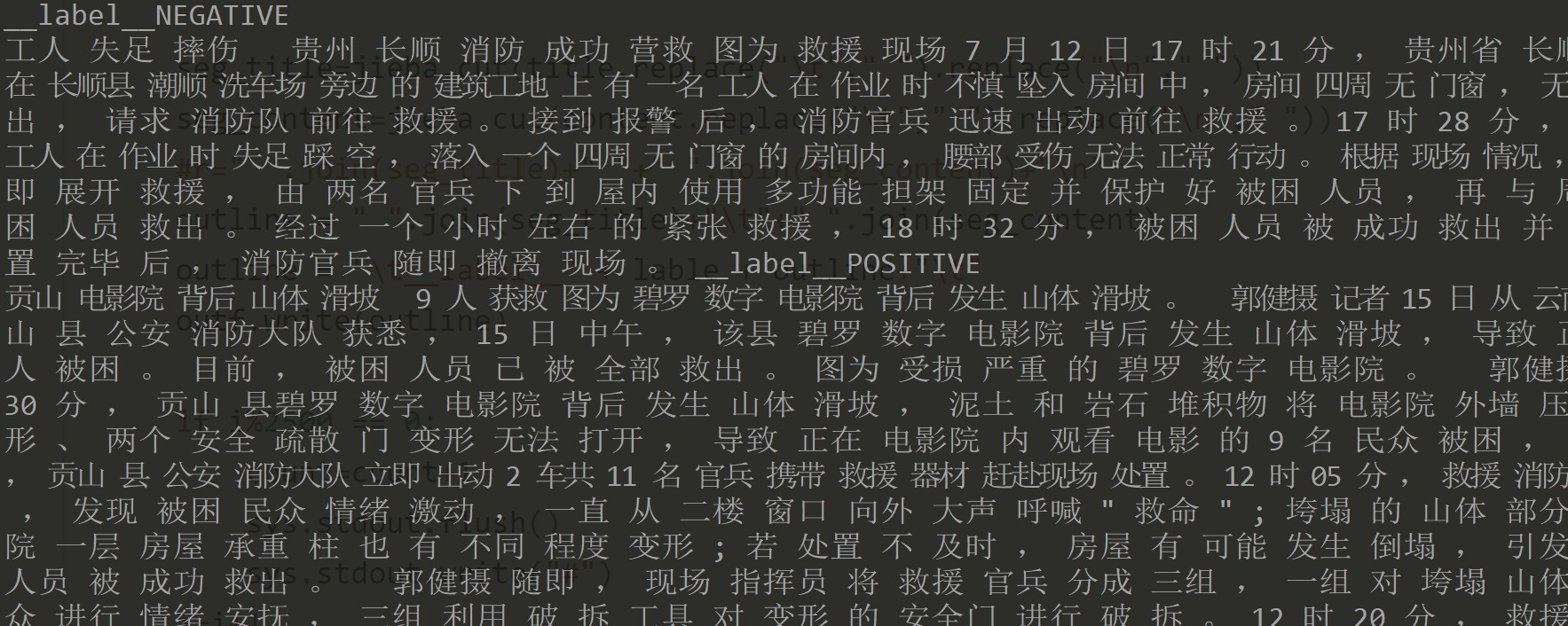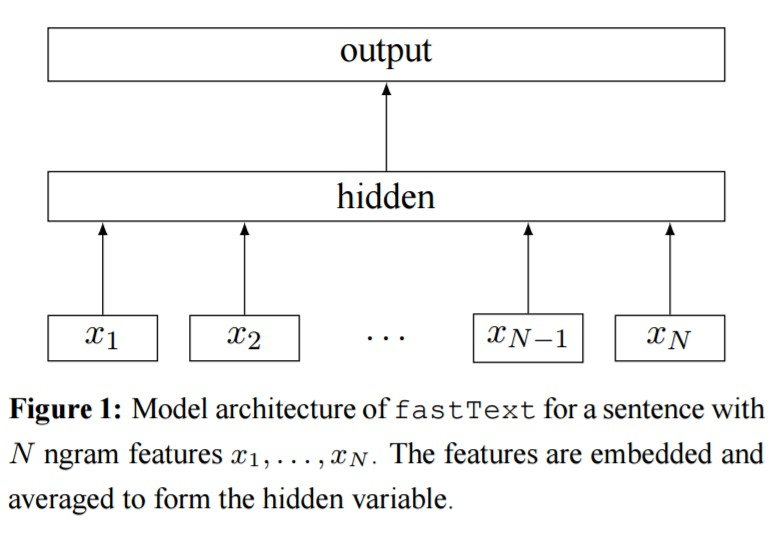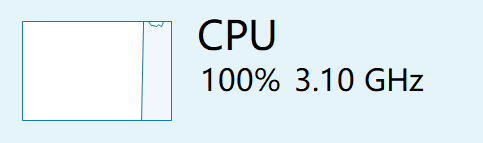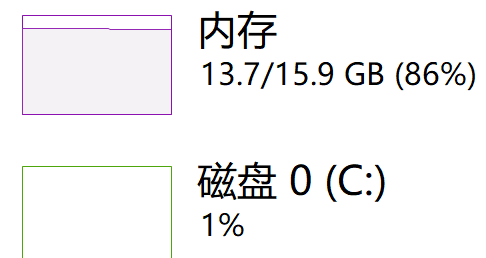# 使用fasttext实现文本处理及文本预测

### 问题分析

360搜索出的这道题，题目是“360搜索-AlphaGo之后“人机大战”Round 2 ——机器写作与人类写作的巅峰对决”，乍一看挺吓人的，其实是让开发者通过一套模型，来识别一篇文章是机器写出来的，还是人类写出来的。其实是有一定区分的，比如说人类写出来的文章，文章的标题和内容契合度比较高（排除标题党的情况），而且文章正文有一定的逻辑连续性，很少在文章的body中出现乱码。机器写出来的文章在以上方面和人类写出来的文章会有不同之处。

1、存在反复，且不需要反复强调的文字，例如“忌水性物质有生石灰”；

2、逻辑不通顺，文章结尾一个“四”，不知其所指；

3、文章有明显拼凑痕迹，从“一二三四”几点可以看出是从很多篇文章中剪辑而来，上下文关联性弱。

#### 数据格式

Field Type Description Note

Field Type Description Note

Field Type Description Note

### 数据预处理

#encoding=utf-8
import jieba
#author linxinzhu
seg_list = jieba.cut("这个竞赛真的费时间",cut_all=True)
print "Full Mode:", "/ ".join(seg_list) #全模式

seg_list = jieba.cut("zwq沉迷逛QQ空间，还时不时撩妹",cut_all=False)
print "Default Mode:", "/ ".join(seg_list) #精确模式

seg_list = jieba.cut("测试集好大啊，跑一次要好久") #默认是精确模式
print ", ".join(seg_list)

seg_list = jieba.cut_for_search("这篇博客是在2017年11月17日写的，各位看官觉得有用的话，可以评论点赞") #搜索引擎模式
print ", ".join(seg_list)

PS C:\Users\LinXinzhu\py> python fenci.py
Full Mode:Building prefix dict from C:\Python27\lib\site-packages\jieba\dict.txt ...
Loading model from cache c:\users\linxin~1\appdata\local\temp\jieba.cache
Loading model cost 0.804000139236 seconds.
Prefix dict has been built succesfully.
这个/ 竞赛/ 真的/ 费时/ 费时间/ 时间
Default Mode: zwq/ 沉迷/ 逛/ QQ/ 空间/ ，/ 还/ 时不时/ 撩妹

1、代码开头记得写上编码方式，包括后面的fasttext在编码上也挺麻烦的，不写的话有惊喜哦！

2、jieba.cut返回一个list，所以在做字符串拼接的时候要把list转成string，常用的就是“ ”.join()

##### 符号处理
def go_split(s,min_len):
# 拼接正则表达式
symbol = '，；。！、？!'
symbol = "[" + symbol + "]+"
# 一次性分割字符串
result = re.split(symbol, s)
return [x for x in result if len(x)>min_len]

def is_dup(s,min_len):
result = go_split(s,min_len)
return len(result) !=len(set(result))

def is_neg_symbol(uchar):
neg_symbol=['!', '0', ';', '?', '、', '。', '，']
return uchar in neg_symbol
##### 特殊字处理

if (ur"，的" in s0) and (not(ur"，的确" in s0)) and (not(ur"，的士" in s0)) \
and (not(ur"，的哥" in s0)) and (not(ur"，的的确确" in s0)):
flag = "NEGATIVE"
if (ur"，了" in s0) and (not(ur"，了解" in s0)) and (not(ur"，了结" in s0)) \
and (not(ur"，了无" in s0)) and (not(ur"，了却" in s0)) \
and (not(ur"，了不起" in s0)):
flag = "NEGATIVE"

if (ur"。的" in s0) and (not(ur"。的确" in s0)) and (not(ur"。的士" in s0)) \
and (not(ur"。的哥" in s0)) and (not(ur"。的的确确" in s0)):
flag = "NEGATIVE"
if (ur"。了" in s0) and (not(ur"。了解" in s0)) and (not(ur"。了结" in s0)) \
and (not(ur"。了无" in s0)) and (not(ur"。了却" in s0)) \
and (not(ur"。了不起" in s0)):
flag = "NEGATIVE"

if (ur";的" in s0) and (not(ur";的确" in s0)) and (not(ur";的士" in s0)) \
and (not(ur";的哥" in s0)) and (not(ur";的的确确" in s0)):
flag = "NEGATIVE"
if (ur";了" in s0) and (not(ur"了解;" in s0)) and (not(ur";了结" in s0)) \
and (not(ur";了无" in s0)) and (not(ur";了却" in s0)) \
and (not(ur";了不起" in s0)):
flag = "NEGATIVE"

if (ur"?的" in s0) and (not(ur"?的确" in s0)) and (not(ur"?的士" in s0)) \
and (not(ur"?的哥" in s0)) and (not(ur"?的的确确" in s0)):
flag = "NEGATIVE"
if (ur"?了" in s0) and (not(ur"?了解" in s0)) and (not(ur"?了结" in s0)) \
and (not(ur"?了无" in s0)) and (not(ur"?了却" in s0)) \
and (not(ur"?了不起" in s0)):
flag = "NEGATIVE"

##### 分词并转换成fasttext格式
#encoding=utf-8
#author linxinzhu
import jieba
import sys
reload(sys)
sys.setdefaultencoding('utf8')
i = 0
count=0
f = open("train.tsv", 'r')
#f = open("evaluation_public.tsv", 'r')
outf = open("lab3fenci.csv",'w')
#outf = open("lab3fencitest.csv",'w')

for line in f:
r = ""
try:
r = line.decode("UTF-8")
except:
print "charactor code error UTF-8"
pass
if r == "":
try:
r = line.decode("GBK")
except:
print "charactor code error GBK"
pass
line=line.strip()
l_ar=line.split("\t")
if len(l_ar)!=4:
continue
id=l_ar
title=l_ar
content=l_ar
lable=l_ar

seg_title=jieba.cut(title.replace("\t"," ").replace("\n"," "))
seg_content=jieba.cut(content.replace("\t"," ").replace("\n"," "))
#r=" ".join(seg_title)+" "+" ".join(seg_content)+"\n"
outline = " ".join(seg_title)+"\t"+" ".join(seg_content)
outline = "\t__label__" + lable + outline+"\t"
outf.write(outline)

if i%2500 == 0:
count=count+1
sys.stdout.flush()
sys.stdout.write("#")
i=i+1

f.close()
outf.close()
print "\nWord segmentation complete."
print i### 模型建立

pip install fasttextfastText的模型架构类似于CBOW，两种模型都是基于Hierarchical Softmax，都是三层架构：输入层、 隐藏层、输出层。
CBOW模型又基于N-gram模型和BOW模型，此模型将W(t−N+1)……W(t−1)作为输入，去预测W(t)
fastText的模型则是将整个文本作为特征去预测文本的类别。

#### 一些比较重要的函数

import fasttext

# Skipgram model
model = fasttext.skipgram('data.txt', 'model')
print model.words # list of words in dictionary

# CBOW model
model = fasttext.cbow('data.txt', 'model')
print model.words # list of words in dictionary

classifier = fasttext.supervised('data.train.txt', 'model')

data.train.txt是一种含有训练句子 每行加上标签的文本文件。默认情况下，假设标签的话， 前缀字符串__label__

result = classifier.test('test.txt')
print 'P@1:', result.precision
print 'R@1:', result.recall
print 'Number of examples:', result.nexamples

texts = ['example very long text 1', 'example very longtext 2']
labels = classifier.predict(texts)
print labels

# Or with the probability
labels = classifier.predict_proba(texts)
print labels

input_file     training file path (required)
output         output file path (required)
lr             learning rate [0.05]
lr_update_rate change the rate of updates for the learning rate 
dim            size of word vectors 
ws             size of the context window 
epoch          number of epochs 
min_count      minimal number of word occurences 
neg            number of negatives sampled 
word_ngrams    max length of word ngram 
loss           loss function {ns, hs, softmax} [ns]
bucket         number of buckets 
minn           min length of char ngram 
maxn           max length of char ngram 
thread         number of threads 
t              sampling threshold [0.0001]
silent         disable the log output from the C++ extension 
encoding       specify input_file encoding [utf-8]

model = fasttext.skipgram('train.txt', 'model', lr=0.1, dim=300)

##### 分类器的属性和方法
classifier.labels                  # List of labels
classifier.label_prefix            # Prefix of the label
classifier.dim                     # Size of word vector
classifier.ws                      # Size of context window
classifier.epoch                   # Number of epochs
classifier.min_count               # Minimal number of word occurences
classifier.neg                     # Number of negative sampled
classifier.word_ngrams             # Max length of word ngram
classifier.loss_name               # Loss function name
classifier.bucket                  # Number of buckets
classifier.minn                    # Min length of char ngram
classifier.maxn                    # Max length of char ngram
classifier.lr_update_rate          # Rate of updates for the learning rate
classifier.t                       # Value of sampling threshold
classifier.encoding                # Encoding that used by classifier
classifier.test(filename, k)       # Test the classifier
classifier.predict(texts, k)       # Predict the most likely label
classifier.predict_proba(texts, k) # Predict the most likely label include their probability

### 调试分析

classifier = fasttext.supervised("lab3fenci.csv","lab3fenci.model",label_prefix="__label__")

result = classifier.test("lab3fenci.csv")
print result.precision
print result.recall

texts = ['它被誉为"天下第一果"，补益气血，养阴生津，现在吃正应季!  六七月是桃

labels = classifier.predict(li)
print labels

classifier = fasttext.supervised("lab3fenci.csv","lab3fenci.model",label_prefix="__label__",lr=0.1,epoch=100,dim=200,bucket=5000000)
result = classifier.test("lab3fenci.csv")
print result.precision
print result.recallclassifier = fasttext.load_model('lab3fenci.model.bin', label_prefix='__label__')

# _*_coding:utf-8 _*_
import fasttext
#author linxinzhu
#load训练好的模型
classifier = fasttext.load_model('lab3fenci.model.bin', label_prefix='__label__')

i=0
f = open("evaluation_public.tsv", 'r')
outf = open("sub.csv",'w')
for line in f:
outline=""
if i==400000:
break
r = ""
try:
r = line.decode("UTF-8")
except:
print "charactor code error UTF-8"
pass
if r == "":
try:
r = line.decode("GBK")
except:
print "charactor code error GBK"
pass
line=line.strip()
l_ar=line.split("\t")
id=l_ar
title=l_ar
content=l_ar
s=""
li=[]
li=list()
s="".join(content)
li=s.split("")
texts=li
labels = classifier.predict(li)
#print id
#print labels
strlabel=str(labels)
if strlabel=="POSITIVE" :
outline = id+"," + "POSITIVE" + "\n"
outf.write(outline)
if strlabel=="NEGATIVE" :
outline = id+"," + "NEGATIVE" + "\n"
outf.write(outline)
i=i+1
del s
del li
f.close()
outf.close()

### 提交结果（截至2017年11月16日）Email： linxinzhulxz@gmail.com